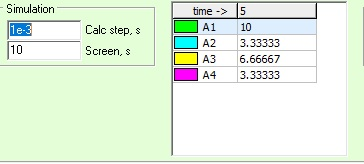∑iin=∑iout

“>iin=iout:  sum of current going into a node = sum of current going out of the same node

Now we add a resistor in series to change the currentSo now A2 and A4 are in series so the resistors add together to 100Ω

A3: 10A * (  A24 / ( A24 + A3) ) = 10A * 100Ω / 150Ω = 6.6667A

A2 and A4 would have the same current flowing through both of them since they are in series.

= 10A * A3 / (A24 + A3 )  = 10A * 50Ω / ( 100Ω + 50Ω ) = 3.333A for A2 and A4.

10A = A2 + A3 = 3.3333A + 6.6667A